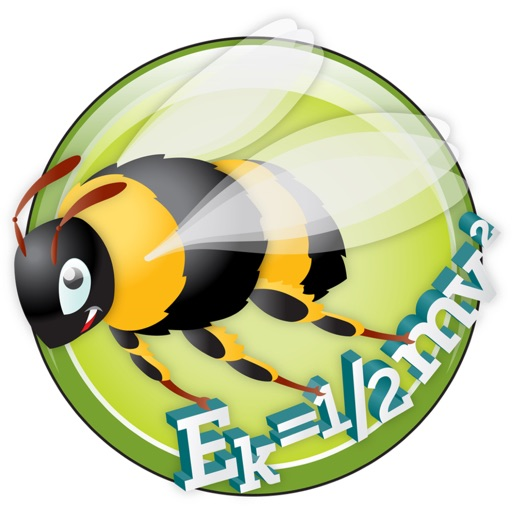## EquationLab supports and inspires students, parents and teachers in all areas of formula calculation in high school Physics and Maths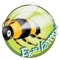# EquationLab

by Go Think Learning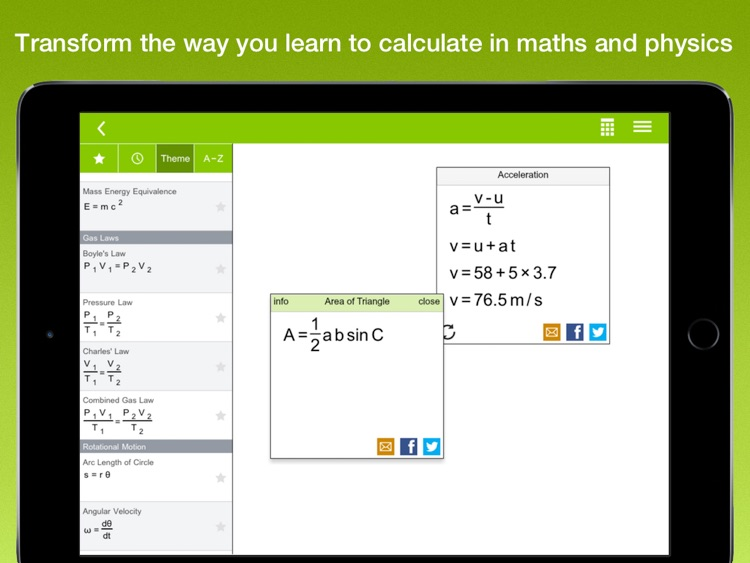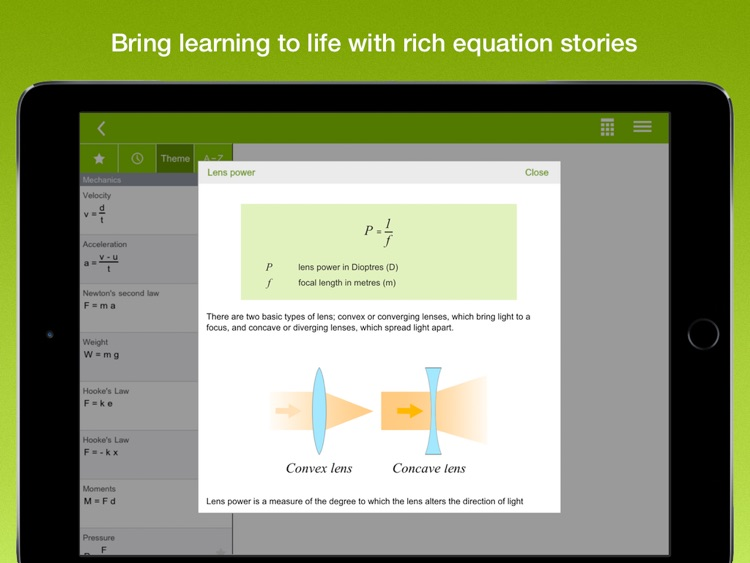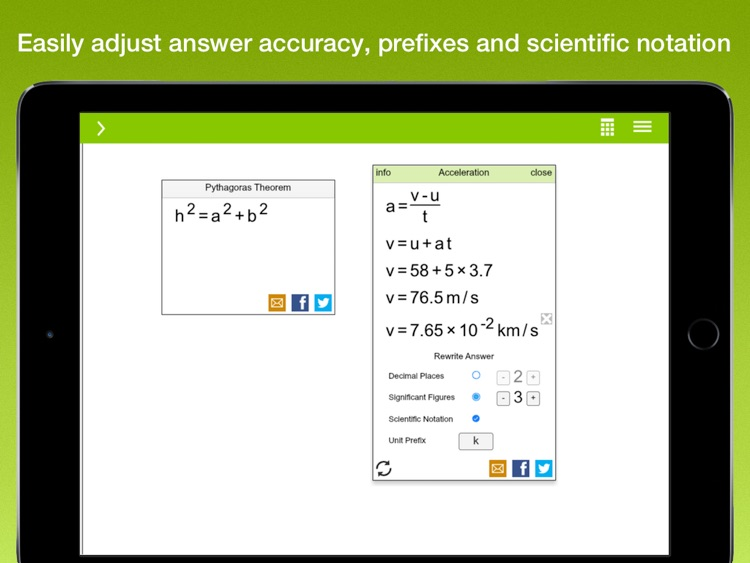EquationLab supports and inspires students, parents and teachers in all areas of formula calculation in high school Physics and Maths.### App Details

Version
2.2.52
Rating
NA
Size
64Mb
Genre
Education Productivity
Last updated
May 30, 2020
Release date
August 27, 2015

### EquationLab is FREE but there are more add-ons

• \$11.99

Physics and Maths equation set

### App Screenshots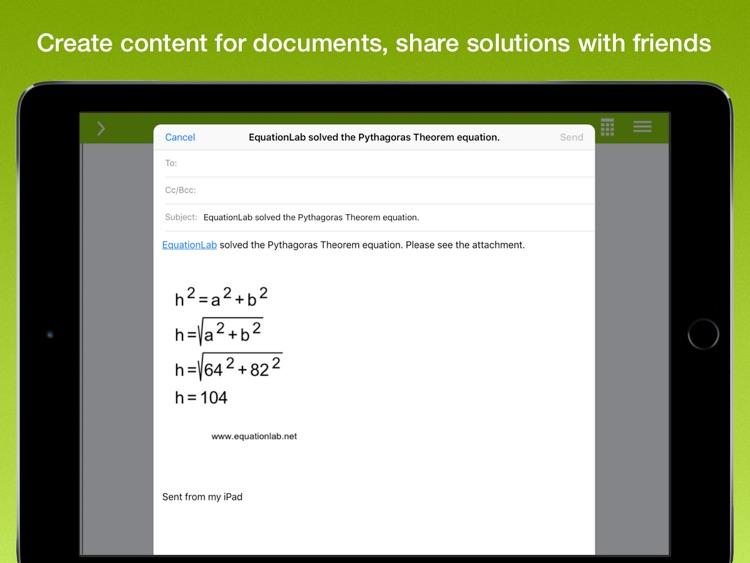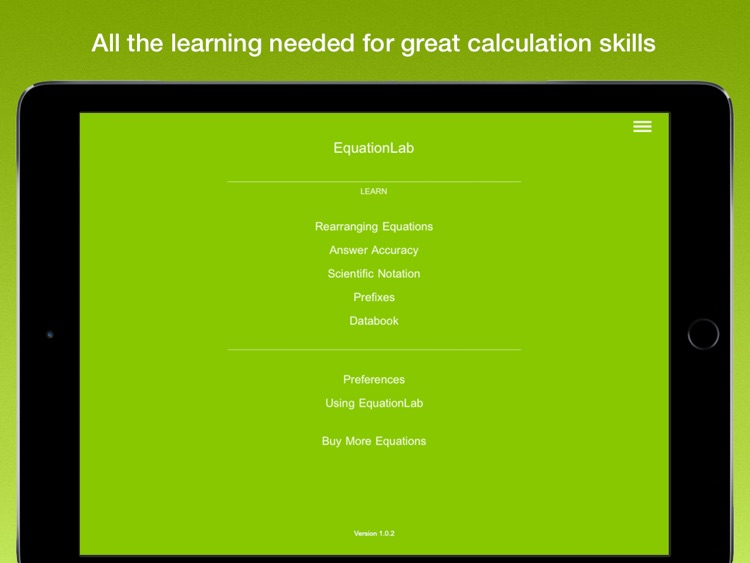### App Store Description

EquationLab supports and inspires students, parents and teachers in all areas of formula calculation in high school Physics and Maths.

Students:
• Easily solve equation based calculations for homework and class - everything you need is in the app.
• Check your physics and maths homework is correct - even when you need to rearrange equations.
• Learn, practice and interact with all the important equation concepts you need to succeed in high school Maths and Physics.
• Model your written work on EquationLab's solutions.
• Share your calculations with teachers and friends.

Parents:
• Check your child's homework is correct without having to remember how to do formula calculations.
• Give your child a resource to support and enrich learning at home.
• Help your child engage with maths and physics in a way which is fast, fun and interactive.

Teachers:
• Enrich classwork and engage students with a ready made formula calculation resource.
• Save time and boost your productivity by creating perfectly laid out calculation resources for documents and presentations.

** EquationLab **

Designed by experienced educators, EquationLab enriches a wide range of learning experiences where formula calculation is the focus, inspiring students to engage with this important area. It reinforces an effective calculation process and helps students become secure in their calculation skills. It's also a great productivity tool which saves valuable time when producing equation resources for documents and presentations.

Fast, intuitive drag and drop solving makes calculation easy:
• Select an equation, drag and drop to re-arrange, tap in values and get the answer - it's easy to solve any formula based calculation.
• Get solutions in a fraction of the time it takes with pen, paper and calculator.
• Rearrange your equation and enter values, or enter values in equations first and then rearrange, it's up to you. The latter approach is favoured by some examination boards. EquationLab lets you work both ways.

Rich, colourful equation stories:
• Equation explanations and sample calculations show what each physics and maths equation is used for so you can learn while you calculate.

Share perfectly laid out calculations - in seconds:
• Create perfect solutions and easily drop into documents or presentations.
• Save calculation graphics to your iPad, export to other applications, or share on social media with friends and colleagues.

Interactive algebra tutor:
• Learning to rearrange equations can be a significant hurdle for many young people. Practising rearranging equations using EquationLab's powerful algebra tutor is a fun and engaging way for students to learn.

Prefixes, decimal places, significant figures and scientific notation:
• Interact and understand these important concepts as you alter the way your input values and answers are displayed.

Text book content covers all important equation concepts:
• In app chapters on rearranging equations, prefixes, decimal places, significant figures and scientific notation.
• A data book lets you quickly look up all important constants so you can use them in your calcuations.

Scientific calculator:
• Students will need a calculator in exams, so the app has one.

** In App Purchase **

EquationLab can be downloaded free with 6 sample equations. An In App Purchase gives you the full version of the app which contains almost 100 equations, equation stories and unlocks EquationLab's powerful interactive algebra tutor. Having completed the purchase, you will automatically get all future upgrades, for life!

The full equation set includes:

• Maths: Fractions, percentages, circumferences and perimeters, areas, volumes, circle arc and sector, Pythagoras, line and gradient, trigonometry, sine and cosine rule, trigonometric area, roots of a quadratic equation.

• Physics: Mechanics, heat, waves, electricity, power and efficiency, optics, nuclear physics, gas laws and rotational motion.

Disclaimer:
AppAdvice does not own this application and only provides images and links contained in the iTunes Search API, to help our users find the best apps to download. If you are the developer of this app and would like your information removed, please send a request to [email protected] and your information will be removed.